X

Скопируйте код и вставьте его на свой сайт.

Ширина px

Вы можете уменьшить размер презентации, указав свой размер!

# 6786876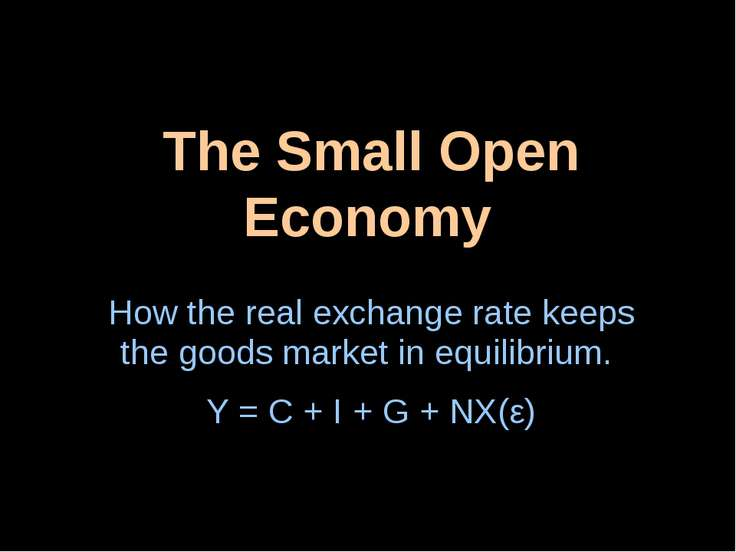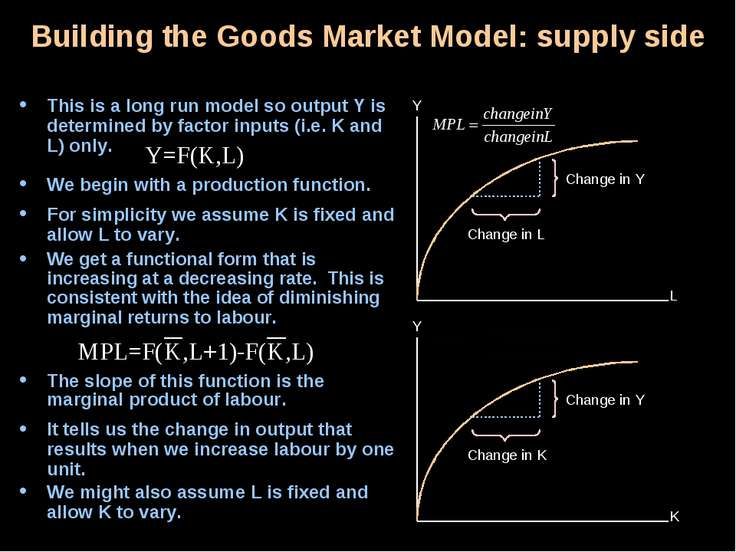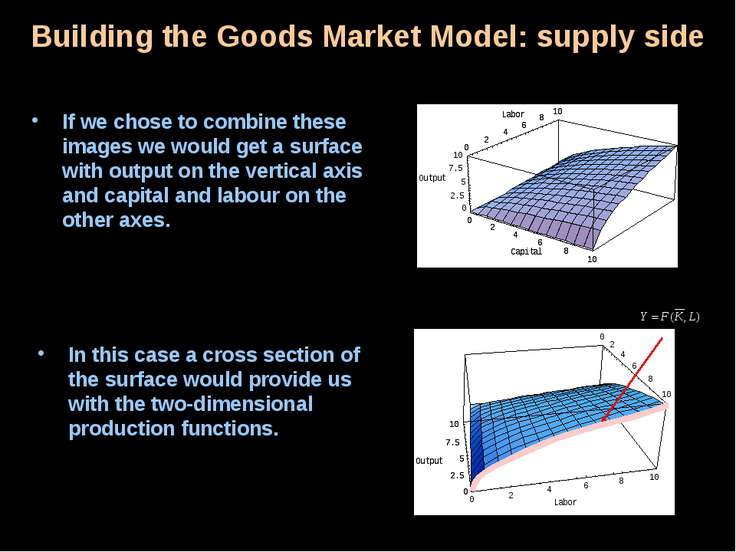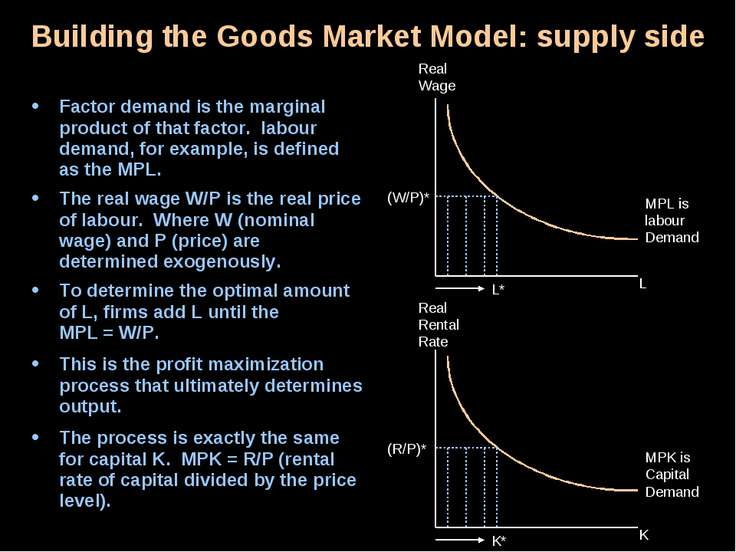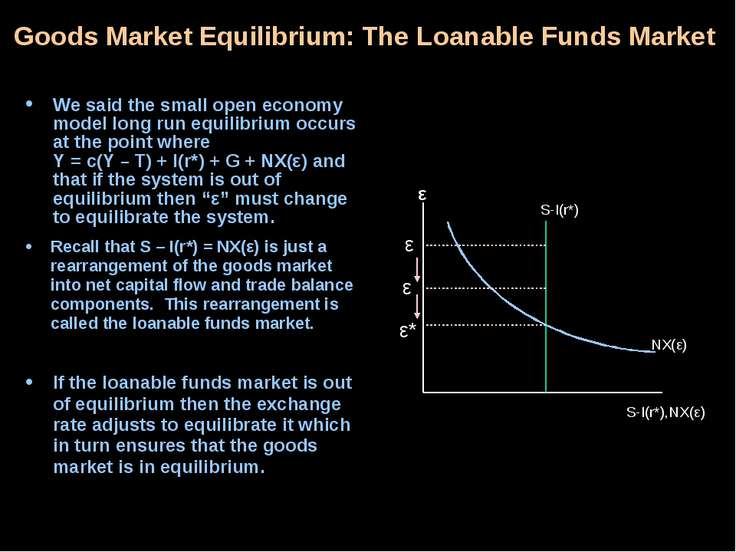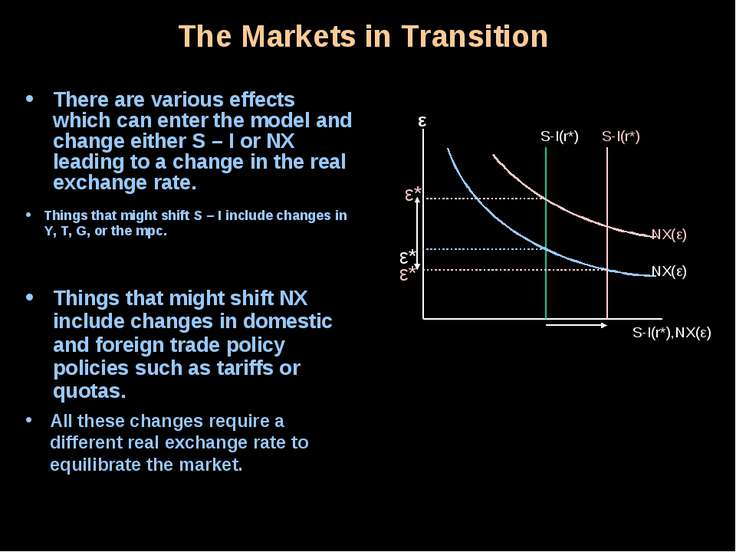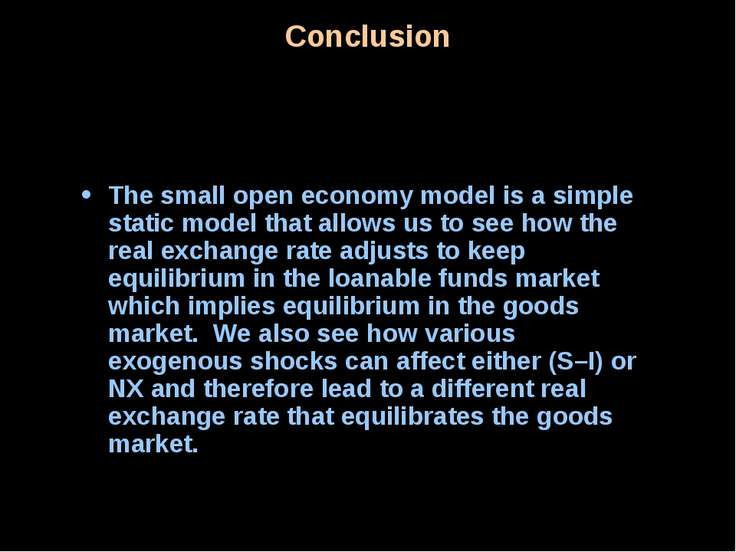Класс
Автор

6786876

Описание презентации по отдельным слайдам:

1 слайд

The Small Open Economy How the real exchange rate keeps the goods market in equilibrium. Y = C + I + G + NX(ε)

2 слайд

Model Background This model is open in the sense that there are exports (X) and imports (M) in the model. Note that net exports (NX) equals (X–M). The model still includes government tax and expenditure. The real exchange rate (not the interest rate) is the equilibrating force in this model. In other words if the model is out of equilibrium it is the changing real exchange rate that returns the model to equilibrium. Y > C + I + G + NX(ε) => exchange rate decreases => NX increases until Y = C + I + G + NX(ε) Y = C + I + G + NX(ε) The left hand side of the goods market represents supply The right hand side represents demand. Y < C + I + G + NX(ε) => exchange rate increases => NX decreases until Y = C + I + G + NX(ε)

3 слайд

Building the Goods Market Model: supply side This is a long run model so output Y is determined by factor inputs (i.e. K and L) only. Change in Y Change in L Change in Y Change in K We begin with a production function. For simplicity we assume K is fixed and allow L to vary. The slope of this function is the marginal product of labour. It tells us the change in output that results when we increase labour by one unit. We might also assume L is fixed and allow K to vary. We get a functional form that is increasing at a decreasing rate. This is consistent with the idea of diminishing marginal returns to labour.

4 слайд

Building the Goods Market Model: supply side If we chose to combine these images we would get a surface with output on the vertical axis and capital and labour on the other axes. In this case a cross section of the surface would provide us with the two-dimensional production functions.

5 слайд

Building the Goods Market Model: supply side Factor demand is the marginal product of that factor. labour demand, for example, is defined as the MPL. MPL is labour Demand (W/P)* L* (R/P)* K* MPK is Capital Demand The real wage W/P is the real price of labour. Where W (nominal wage) and P (price) are determined exogenously. To determine the optimal amount of L, firms add L until the MPL = W/P. This is the profit maximization process that ultimately determines output. The process is exactly the same for capital K. MPK = R/P (rental rate of capital divided by the price level).

6 слайд

Building the Goods Market Model: demand side We begin with consumption, investment, government expenditure, and net exports. This gives us the following national income accounting identity. Y = C + I(r*) + G + NX(ε) …We know Y=F(K,L) Now, given a savings rate “s” we say c = (1–s) is the marginal propensity to consume. This gives us a consumption function C = c(Y–T). “r” is the real interest rate. Investment and the real interest rate have a negative relationship so I(r) is negatively sloped. As “r” increases “I” decreases. In this case however it is the world interest rate (r*) that dominates the small open economy. Much like a perfect competitor is a price taker the small open economy is an interest rate taker. Domestic investors always have access to the world interest rates and their economy is so small it can not affect the world interest rate. “T” is the amount of tax collected. NX = (X–M) is the trade balance and is dependent on the real exchange rate “ε” From this we get… Y = c(Y–T) + I(r*) + G + NX(ε) …rearranging we get, Y – c(Y–T) – G = I(r*) + NX(ε) …or, Sn = I(r*) + NX(ε) …or Sn – I(r*) = NX(ε) …so national savings – investment = net exports we call (S–I) net capital outflow because when savings is positive it is lent abroad.

7 слайд

Goods Market Equilibrium: The Loanable Funds Market We said the small open economy model long run equilibrium occurs at the point where Y = c(Y – T) + I(r*) + G + NX(ε) and that if the system is out of equilibrium then “ε” must change to equilibrate the system. S-I(r*),NX(ε) ε ε* S-I(r*) NX(ε) ε ε Recall that S – I(r*) = NX(ε) is just a rearrangement of the goods market into net capital flow and trade balance components. This rearrangement is called the loanable funds market. If the loanable funds market is out of equilibrium then the exchange rate adjusts to equilibrate it which in turn ensures that the goods market is in equilibrium.

8 слайд

The Markets in Transition There are various effects which can enter the model and change either S – I or NX leading to a change in the real exchange rate. Things that might shift S – I include changes in Y, T, G, or the mpc. Things that might shift NX include changes in domestic and foreign trade policy policies such as tariffs or quotas. S-I(r*),NX(ε) ε ε* S-I(r*) NX(ε) ε* ε* NX(ε) S-I(r*) All these changes require a different real exchange rate to equilibrate the market.

9 слайд

Conclusion The small open economy model is a simple static model that allows us to see how the real exchange rate adjusts to keep equilibrium in the loanable funds market which implies equilibrium in the goods market. We also see how various exogenous shocks can affect either (S–I) or NX and therefore lead to a different real exchange rate that equilibrates the goods market.